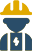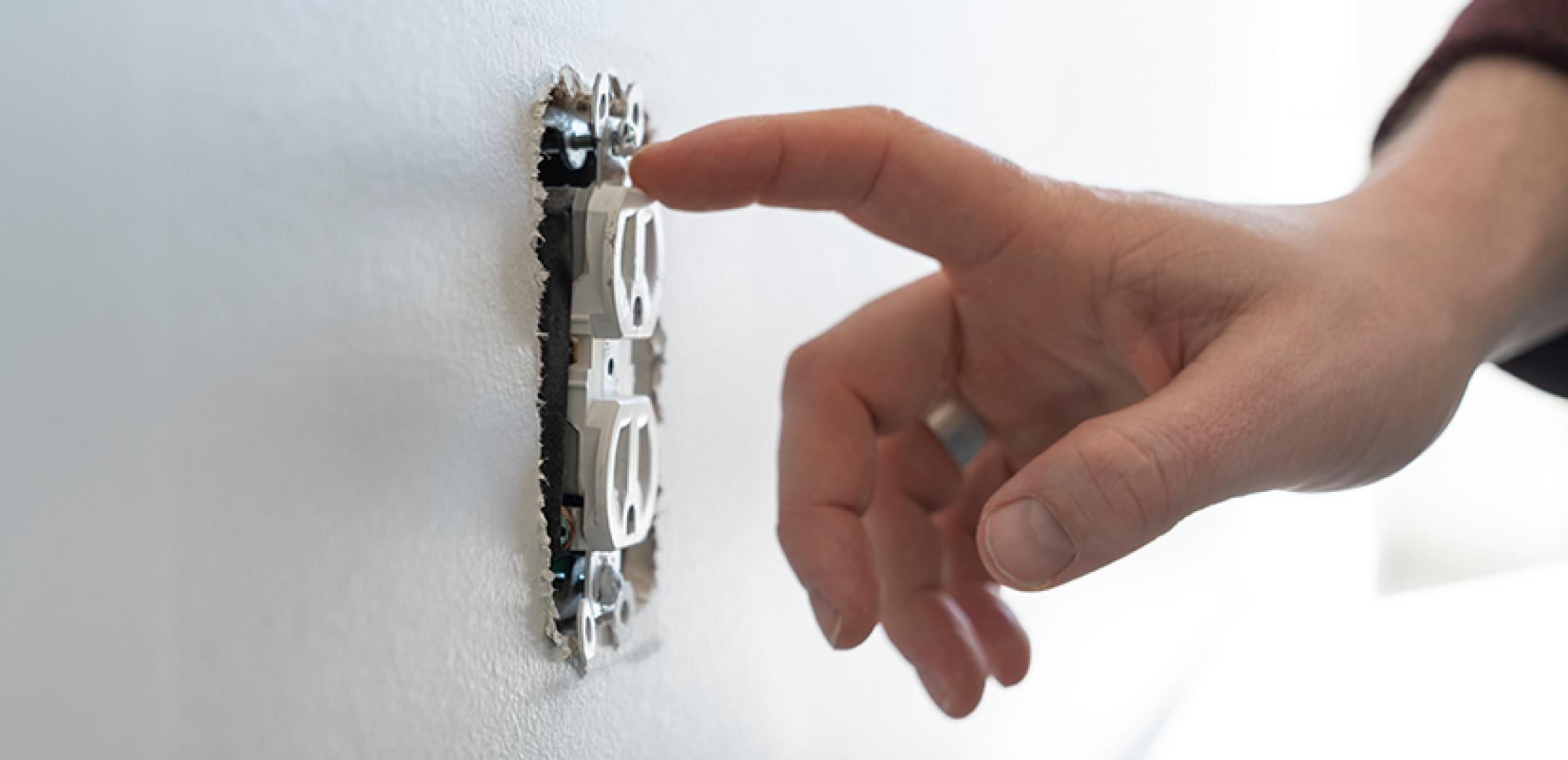Call This

Monday

to Get

\$35 OFFCall Us Now

#### Recent Blogs#### Our Services

•Electrical Code Corrections
•Electric Meter Box
•Lighting Installation

#### Tags

How to Measure PowerFebruary 01, 2023

# How to Measure Power

Electricity: such a pervasive yet little understood concept. We all think we know what electricity does: gives power and zaps people. But few of us understand the technical intricacies behind this ubiquitous phenomenon. Most simply, electricity is generated by the movement of a loop of wire between the poles of a magnet. The device that converts potential and kinetic energy into electricity is called a generator. On the macro level, electricity is produced from electrical power plants via turbines and other machines such as engines. Well over half of the electricity generated in the United States is done through the use of steam turbines. The following information on electrical power is brought to you by Neighborhood Electric.

Some Terms

To understand the relationship of the following terms, let us start with the basic equation that is Ohms Law:

I = V/R (Current is equal to Voltage divided by Resistance)

The current is measured in amps while voltage is measured in volts and resistance is measured in ohms. The relationship between these are often explained using the analogy of water pipes. The voltage can be compared to the water pressure, the current is equal to the flow rate, and the resistance is the equivalent of the pipes diameter.

Lets imagine you have a hose that is an inch in diameter connected to a water tank. If you increase the pressure of the water tank then surely the water will come out of the hose with more force. Similarly, increasing the voltage in an electrical system will increase the current flow. If, on the other hand, you attach a hose that is 2 inches in diameter to the same water tank, then you will also have more water come out in the same time span. Likewise, decreasing the resistance in an electrical system increases the current flow. Essentially, the current has a direct relationship with the voltage and an inverse relationship with the resistance.

There are two types of currents: Direct and Alternating. Youve probably heard them described as DC vs AC. In a direct current, electricity flows in one direction. An alternating current has electricity changing directions of its flow periodically. Most electronics use DC while houses are wired for AC.

## How is Electricity Measured?

In honor of the inventor of the steam engine, James Watt, the unit of measurement for electric power is the Watt. Though some devices are capable of operating using only a few Watts, a more complex and large devices power consumption is measured using kilowatts. If you have taken a look at your meter then youve probably seen that your utility provider measures your usage in kWh (kilowatthours). A kWh is the energy of a kilowatt supplied via an electric circuit for an hour.

In short, amperage (current) multiplied by the voltage is equal to the wattage. If you know your systems amperage and understand how many volts your outlet can produce, then you can calculate the maximum amount of watts your circuit can support at one time by multiplying the two. Make sure that the amps multiplied by the voltage does not exceed the wattage. Exceeding that amount risks an electrical blowout.